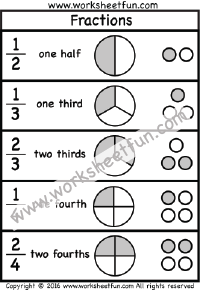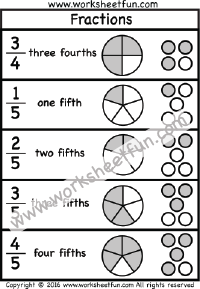Fractions – Word Form – Number Form – Halves, Thirds, Fourths, Fifths – Two Worksheets

Fractions – Word Form – Number Form – Halves, Thirds, Fourths, Fifths – Two WorksheetsFraction

Fraction Circles

Fraction Circles Template

Fraction Model

Fraction Subtraction

Fractions – Coloring

Fractions – Comparing

Fractions – Equivalent